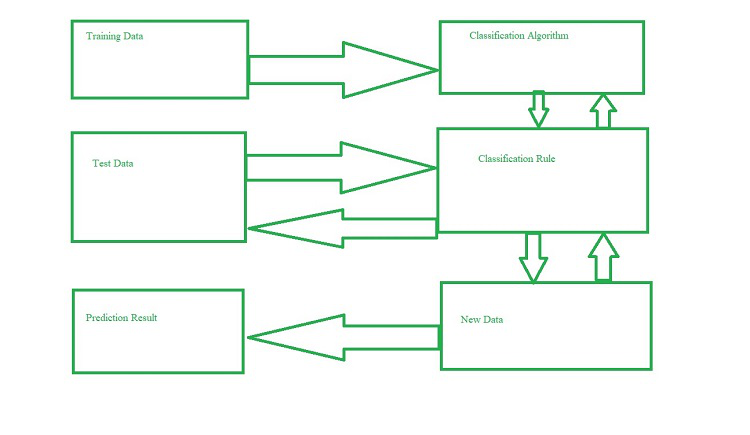# What is Prediction in Data Mining?

• Difficulty Level : Hard
• Last Updated : 17 Mar, 2022

To find a numerical output, prediction is used. The training dataset contains the inputs and numerical output values. According to the training dataset, the algorithm generates a model or predictor. When fresh data is provided, the model should find a numerical output. This approach, unlike classification, does not have a class label. A continuous-valued function or ordered value is predicted by the model.

In most cases, regression is utilized to make predictions.  For example: Predicting the worth of a home based on facts like the number of rooms, total area, and so on.

Consider the following scenario: A marketing manager needs to forecast how much a specific consumer will spend during a sale. In this scenario, we are bothered to forecast a numerical value. In this situation, a model or predictor that forecasts a continuous or ordered value function will be built.### Prediction Issues:

Preparing the data for prediction is the most pressing challenge. The following activities are involved in data preparation:

• Data Cleaning: Cleaning data include reducing noise and treating missing values. Smoothing techniques remove noise, and the problem of missing values is solved by replacing a missing value with the most often occurring value for that characteristic.
• Relevance Analysis: The irrelevant attributes may also be present in the database. The correlation analysis method is used to determine whether two attributes are connected.
• Data Transformation and Reduction:  Any of the methods listed below can be used to transform the data.
• Normalization: Normalization is used to transform the data. Normalization is the process of scaling all values for a given attribute so that they lie within a narrow range. When neural networks or methods requiring measurements are utilized in the learning process, normalization is performed.
• Generalization: The data can also be modified by applying a higher idea to it. We can use the concept of hierarchies for this.

Other data reduction techniques include wavelet processing, binning, histogram analysis, and clustering.

My Personal Notes arrow_drop_up